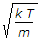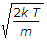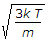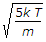# Mechanical Engineering - Thermodynamics

### Exercise :: Thermodynamics - Section 1

16.

The most probable velocity of the gas molecules is given by

 A.B.C.D.Explanation:

No answer description available for this question. Let us discuss.

17.

The efficiency of Diesel cycle approaches to Otto cycle efficiency when

 A. cut-off is increased B. cut-off is decreased C. cut-off is zero D. cut-off is constant

Explanation:

No answer description available for this question. Let us discuss.

18.

The entropy __________ in an irreversible cyclic process.

 A. remains constant B. decreases C. increases

Explanation:

No answer description available for this question. Let us discuss.

19.

The atomic mass of oxygen is

 A. 12 B. 14 C. 16 D. 32

Explanation:

No answer description available for this question. Let us discuss.

20.

The ratio of specific heat at constant pressure (cp) and specific heat at constant volume (cv) is

 A. equal to one B. less than one C. greater than one D. none of these